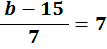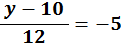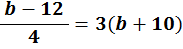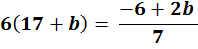### Questions − Solving Equations 2

 1 If   42 + y = 20 + 3y   solve y

 2 If   6 + 4b = 15 + b   solve b

 3 If   37 + t = 2(26 + 3t)   what is the value of t?

 4 If   2(9 + y) = 4(7 + 3y)   what is the value of y?

 5 If   3(y − 120) = 5(y + 30)   what is the value of y?

 6 Ifwhat is the value of b?

 7 Ifwhat is the value of y?

 8 Ifwhat is the value of b?

 9 Ifwhat is the value of b?back to:to: